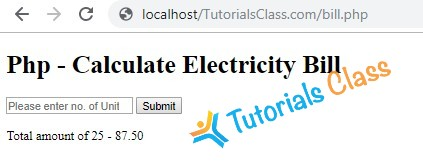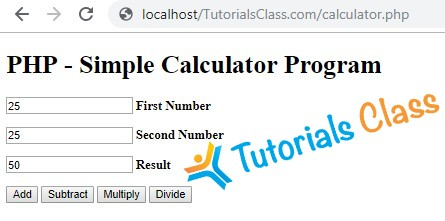# PHP Top Exercises

List of Top PHP Exercise & Assignment for Students. These best & popular exercises help you to understand different logics of PHP Programming. Practice Exercises for PHP Loops, Arrays, Functions, Decision Making, File Handling, Forms & Database Handling.

## Write a factorial program using for loop in php

Description:

Write a program to calculate factorial of a number using for loop in php.

``````<?php
\$num = 3;
\$factorial = 1;

for (\$x=\$num; \$x>=1; \$x--)
{
\$factorial = \$factorial * \$x;
}

echo "The factorial of \$num is \$factorial";
?>``````
Tutorials Class - Output Window

The factorial of 3 is 6

## Write a program to create Chess board in PHP using for loop

Description:

Write a PHP program using nested for loop that creates a chess board.

Conditions:

• You can use html table having width=”400px” and take “30px” as cell height and width for check boxes.

View Solution/Program

``````<table width="400px" cellspacing="0px" cellpadding="0px" border="1px">
<?php
for(\$row=1;\$row<=8;\$row++)
{
echo "<tr>";
for(\$column=1;\$column<=8;\$column++)
{
\$total=\$row+\$column;
if(\$total%2==0)
{
echo "<td height=35px width=30px bgcolor=#FFFFFF></td>";
}
else
{
echo "<td height=35px width=30px bgcolor=#000000></td>";
}
}
echo "</tr>";
}
?>
</table>``````
Tutorials Class - Output Window## Write a program to calculate Electricity bill in PHP

Description:

You need to write a PHP program to calculate electricity bill using if-else conditions.

Conditions:

• For first 50 units – Rs. 3.50/unit
• For next 100 units – Rs. 4.00/unit
• For next 100 units – Rs. 5.20/unit
• For units above 250 – Rs. 6.50/unit
• You can use conditional statements.

View Solution/Program

``````<!DOCTYPE html>

<title>PHP - Calculate Electricity Bill</title>

<?php
\$result_str = \$result = '';
if (isset(\$_POST['unit-submit'])) {
\$units = \$_POST['units'];
if (!empty(\$units)) {
\$result = calculate_bill(\$units);
\$result_str = 'Total amount of ' . \$units . ' - ' . \$result;
}
}
/**
* To calculate electricity bill as per unit cost
*/
function calculate_bill(\$units) {
\$unit_cost_first = 3.50;
\$unit_cost_second = 4.00;
\$unit_cost_third = 5.20;
\$unit_cost_fourth = 6.50;

if(\$units <= 50) {
\$bill = \$units * \$unit_cost_first;
}
else if(\$units > 50 && \$units <= 100) {
\$temp = 50 * \$unit_cost_first;
\$remaining_units = \$units - 50;
\$bill = \$temp + (\$remaining_units * \$unit_cost_second);
}
else if(\$units > 100 && \$units <= 200) {
\$temp = (50 * 3.5) + (100 * \$unit_cost_second);
\$remaining_units = \$units - 150;
\$bill = \$temp + (\$remaining_units * \$unit_cost_third);
}
else {
\$temp = (50 * 3.5) + (100 * \$unit_cost_second) + (100 * \$unit_cost_third);
\$remaining_units = \$units - 250;
\$bill = \$temp + (\$remaining_units * \$unit_cost_fourth);
}
return number_format((float)\$bill, 2, '.', '');
}

?>

<body>
<div id="page-wrap">
<h1>Php - Calculate Electricity Bill</h1>

<form action="" method="post" id="quiz-form">
<input type="number" name="units" id="units" placeholder="Please enter no. of Units" />
<input type="submit" name="unit-submit" id="unit-submit" value="Submit" />
</form>

<div>
<?php echo '<br />' . \$result_str; ?>
</div>
</div>
</body>
</html>``````
Tutorials Class - Output Window## Write a simple calculator program in PHP using switch case

Description:

You need to write a simple calculator program in PHP using switch case.

Operations:

• Subtraction
• Multiplication
• Division

View Solution/Program

``````<!DOCTYPE html>

<title>Simple Calculator Program in PHP - Tutorials Class</title>

<?php
\$first_num = \$_POST['first_num'];
\$second_num = \$_POST['second_num'];
\$operator = \$_POST['operator'];
\$result = '';
if (is_numeric(\$first_num) && is_numeric(\$second_num)) {
switch (\$operator) {
\$result = \$first_num + \$second_num;
break;
case "Subtract":
\$result = \$first_num - \$second_num;
break;
case "Multiply":
\$result = \$first_num * \$second_num;
break;
case "Divide":
\$result = \$first_num / \$second_num;
}
}

?>

<body>
<div id="page-wrap">
<h1>PHP - Simple Calculator Program</h1>
<form action="" method="post" id="quiz-form">
<p>
<input type="number" name="first_num" id="first_num" required="required" value="<?php echo \$first_num; ?>" /> <b>First Number</b>
</p>
<p>
<input type="number" name="second_num" id="second_num" required="required" value="<?php echo \$second_num; ?>" /> <b>Second Number</b>
</p>
<p>
</p>
<input type="submit" name="operator" value="Subtract" />
<input type="submit" name="operator" value="Multiply" />
<input type="submit" name="operator" value="Divide" />
</form>
</div>
</body>
</html>``````
Tutorials Class - Output Window## Write a PHP program to check whether a number is positive, negative or zero

#### Description:

Write a PHP program to check whether a number is positive, negative or zero.

#### Instructions:

• You can use if else conditions.
• You should use appropriate PHP Operators.
• Also check if it not a numeric value.

View Solution/Program

``````<?php
\$number = 324; // enter any number of your choice here
if (\$number > 0) // condition for positive numbers
{
echo \$number . " is a positive number";
} else if (\$number < 0) // condition for negative number
{
echo \$number . " is a negative number ";
} else if (\$number == 0) // condition for zero
{
echo "You have entered zero";
} else {
echo " please enter a numeric value";
}
?>``````
Tutorials Class - Output Window

324 is a positive number